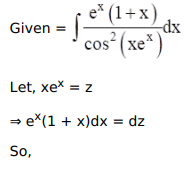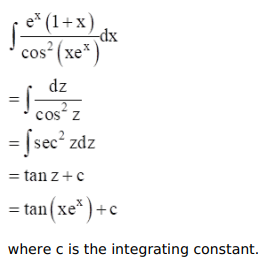# Mark against the correct answer in each of the following:

Question:

Mark $(\sqrt{)}$ against the correct answer in each of the following:

$\int \frac{e^{x}(1+x)}{\cos ^{2}\left(x e^{x}\right)} d x=?$

A. $\tan \left(x e^{x}\right)+C$

B. $\cot \left(x e^{x}\right)+C$

C. $e^{x} \tan x+C$

D. none of these

Solution: